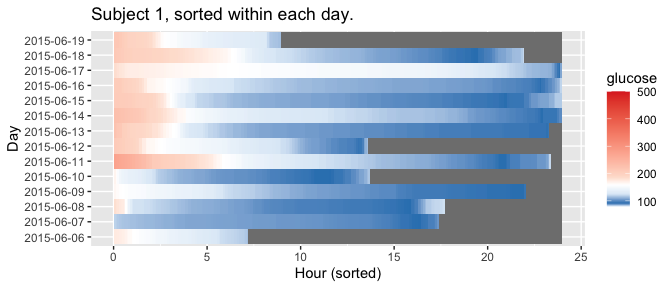# Lasagna Plots in iglu

The plot_glu function supports lasagna plots by changing the ‘plottype’ parameter. For more on lasagna plots, see Swihart et al. (2010) “Lasagna Plots: A Saucy Alternative to Spaghetti Plots.”. The lasagna plots in iglu can be single-subject or multi-subject. The single-subject lasagna plot has rows corresponding to each day of measurements with a color grid indicating glucose values.

The highest glucose values are displayed in red, whereas the lowest are displayed in blue. Thus, the numerical glucose values are mapped to color using the gradient from blue to red, which corresponds to the default ‘blue-red’ color scheme. An alternative ‘red-orange’ color scheme can be selected by the user by corresponding modification of the ‘color_scheme’ parameter.

library(iglu)
plot_glu(example_data_1_subject, plottype = 'lasagna', datatype = "single", tz = 'EST')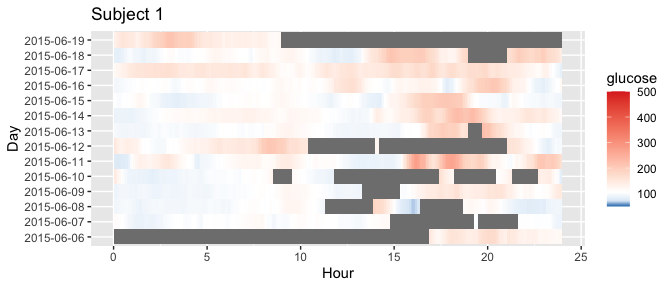plot_glu(example_data_1_subject, plottype = 'lasagna', datatype = "single", color_scheme = "red-orange", tz = 'EST')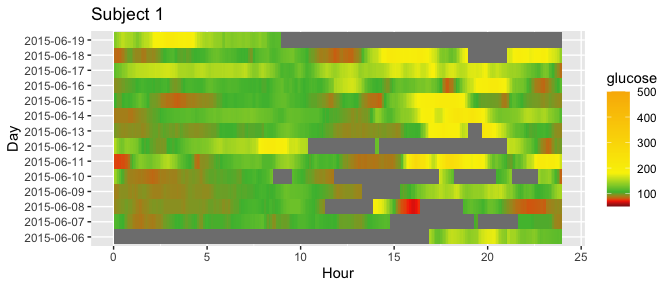For plots with a single subject, setting the datatype parameter to “single” will display a plot where the rows represent days.

plot_glu(example_data_1_subject, datatype = 'single', plottype = 'lasagna', tz = 'EST')We can additionally sort the values at each time point by setting lasagnatype = 'timesorted'

plot_glu(example_data_1_subject, plottype = 'lasagna', datatype = 'single', lasagnatype = 'timesorted', tz = 'EST')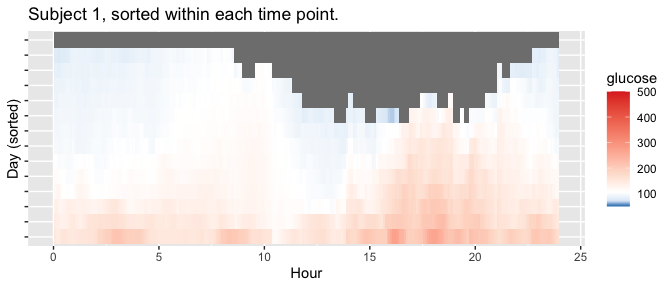To average across days at each time point, we can use datatype = 'average':

plot_glu(example_data_1_subject, plottype = 'lasagna', datatype = 'average', tz = 'EST')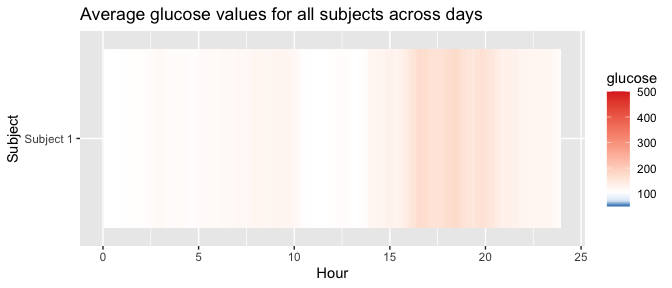You can also produce plots for multiple subjects, by using data with multiple values for “id”. By default, this will produce an unsorted lasagna plot using up to 14 days worth of data with each subject displayed in separate rows.

plot_glu(example_data_5_subject, plottype = 'lasagna', tz = 'EST')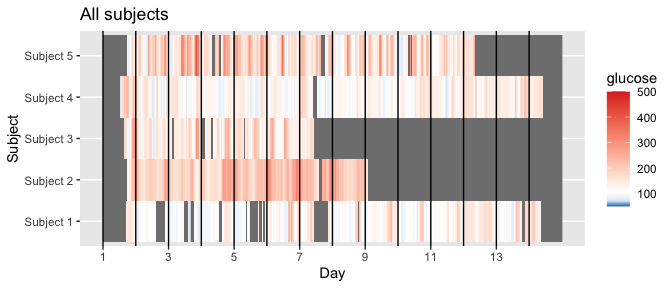This works for all the above options sans datatype = single.

plot_glu(example_data_5_subject, plottype = 'lasagna', color_scheme = "red-orange", tz = 'EST')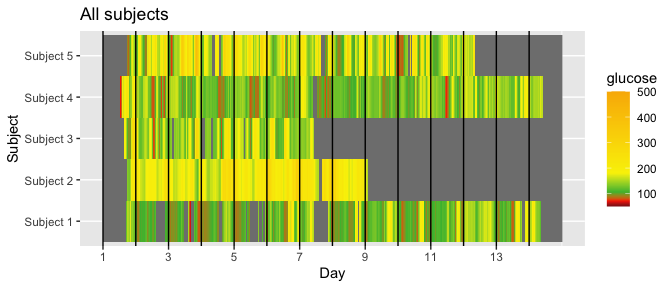plot_glu(example_data_5_subject, plottype = 'lasagna', lasagnatype = 'timesorted', tz = 'EST')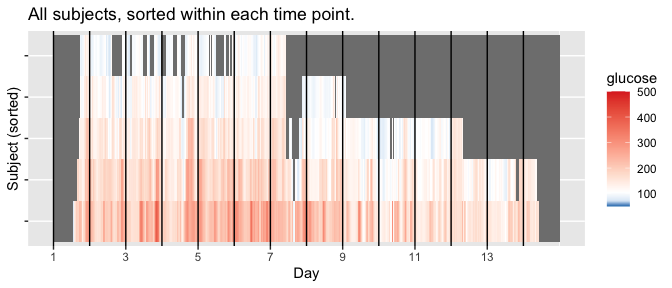plot_glu(example_data_5_subject, plottype = 'lasagna', datatype = 'average', tz = 'EST')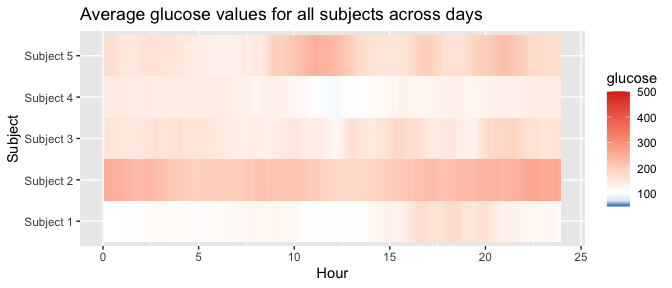Lasagna plots are also an excellent way to visualize gaps in data. The inter_gap parameter controls the maximum length of time (in minutes) between glucose readings for interpolation to occur between them. See how more gaps appear in the plot below as inter_gap shrinks.

plot_glu(example_data_1_subject, plottype ="lasagna", datatype = "single", inter_gap = 150)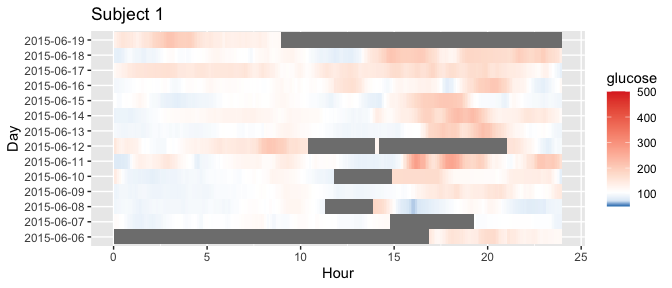plot_glu(example_data_1_subject, plottype ="lasagna", datatype = "single", inter_gap = 45)plot_glu(example_data_1_subject, plottype ="lasagna", datatype = "single", inter_gap = 15)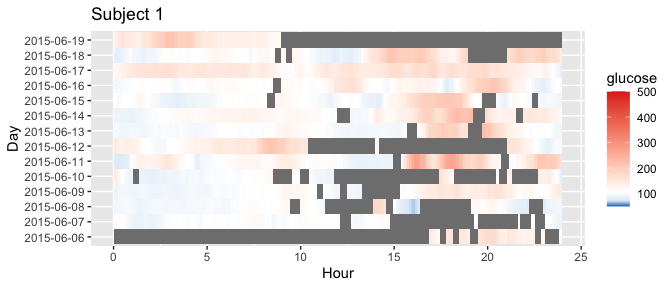For further customization of lasagna plots, use the plot_lasagna and plot_lasagna_1subject functions.

Within plot_lasagna, the “midpoint” parameter specifies the glucose value (in mg/dL) at which the color transitions from blue to red (the default is 105 mg/dL), and the “limit” parameter specifies the range (the default is [50, 500] mg/dL)

plot_lasagna(example_data_5_subject, datatype = "average", midpoint = 140, limits = c(60,400), tz = 'EST')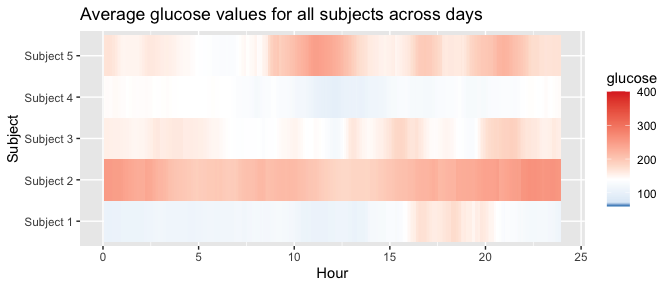plot_lasagna allows for multi-subject lasagna plots with the additional options of sorting the hours by glucose values for each subject, i.e. horizontal sorting, by setting lasagnatype = 'subjectsorted'.

plot_lasagna(example_data_5_subject, datatype = 'average', lasagnatype = 'subjectsorted', tz = 'EST')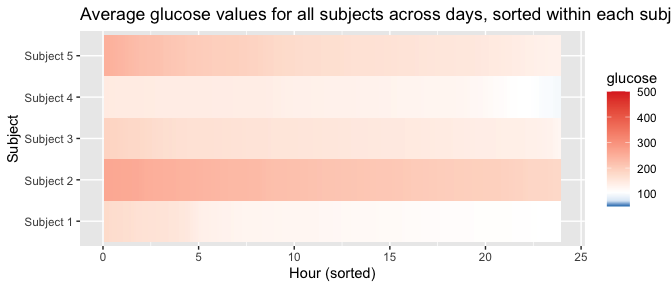plot_lasagna also supports changing the maximum number of days to display, as well as the upper and lower target range limits (LLTR and ULTR), midpoint, and minimum and maximum values to display, all of which will affect the colorbar.

plot_lasagna(example_data_5_subject, datatype = 'average', lasagnatype = 'subjectsorted', LLTR = 100, ULTR = 180, midpoint = 150, limits = c(80, 500), tz = 'EST')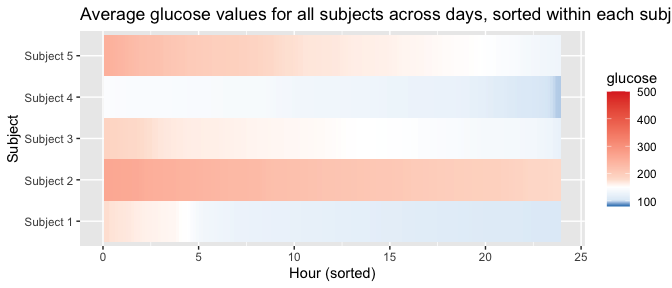plot_lasagna_1subject allows for customization of the more detailed single subject lasagna plots. There is no datatype parameter for plot_lasagna_1subject, but there are three types of plots available, accessed with the lasagnatype parameter.

plot_lasagna_1subject(example_data_1_subject, lasagnatype = 'unsorted', tz = 'EST')plot_lasagna_1subject(example_data_1_subject, lasagnatype = 'timesorted', tz = 'EST')plot_lasagna_1subject(example_data_1_subject, lasagnatype = 'daysorted', tz = 'EST')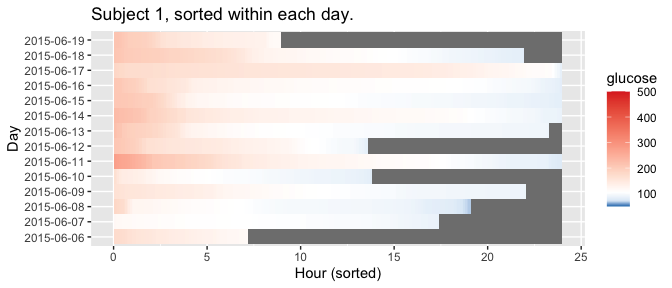As with the lasagna_plot function, changing the LLTR, ULTR, midpoint, and limits parameters will affect the colorbar.

plot_lasagna_1subject(example_data_1_subject, lasagnatype = 'daysorted', midpoint = 150, limits = c(80,500), tz = 'EST')